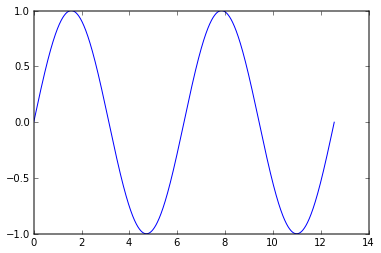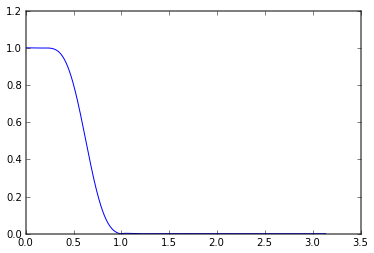# CRÜCIAL PŸTHON: IPython Notebook Demo¶

In :
from IPython.core.display import Image
Image(filename='logo.png', width=600)

Out:## Cells¶

IPython Notebook is a cell-based development environment. Each block of code (or text) can be run independently, and all cells share the same kernel. Each evaluated cell is stored in the In array, and the corresponding output is stored in Out.

Given $a \in \mathbb{R}^D$, compute $\sum_{i = 1}^D a[i]^2$.

In :
def sum_of_squares(a):
return np.sum(a**2)

In :
foo = np.array([1, 3, 5])
sum_of_squares(foo)

Out:
35
In :
print Out + 4

39

In :
# With the --pylab inline option, matplotlib plots are inline as cell output
w = np.linspace(0, 4*pi, 1000)
plt.plot(w, np.sin(w))

Out:
[<matplotlib.lines.Line2D at 0x103d7ec90>]## Tab Completion and documentation¶

IPython will keep track of the members of all of the modules you import and give you tab-completion. It will also tab-complete function arguments and provide help() documentation inline.

In :
import scipy.signal

In [ ]:
scipy.signal.fi

In :
help(scipy.signal.firwin)

Help on function firwin in module scipy.signal.fir_filter_design:

firwin(numtaps, cutoff, width=None, window='hamming', pass_zero=True, scale=True, nyq=1.0)
FIR filter design using the window method.

This function computes the coefficients of a finite impulse response
filter.  The filter will have linear phase; it will be Type I if
numtaps is odd and Type II if numtaps is even.

Type II filters always have zero response at the Nyquist rate, so a
ValueError exception is raised if firwin is called with numtaps even and
having a passband whose right end is at the Nyquist rate.

Parameters
----------
numtaps : int
Length of the filter (number of coefficients, i.e. the filter
order + 1).  numtaps must be even if a passband includes the
Nyquist frequency.

cutoff : float or 1D array_like
Cutoff frequency of filter (expressed in the same units as nyq)
OR an array of cutoff frequencies (that is, band edges). In the
latter case, the frequencies in cutoff should be positive and
monotonically increasing between 0 and nyq.  The values 0 and
nyq must not be included in cutoff.

width : float or None
If width is not None, then assume it is the approximate width
of the transition region (expressed in the same units as nyq)
for use in Kaiser FIR filter design.  In this case, the window
argument is ignored.

window : string or tuple of string and parameter values
Desired window to use. See scipy.signal.get_window for a list
of windows and required parameters.

pass_zero : bool
If True, the gain at the frequency 0 (i.e. the "DC gain") is 1.
Otherwise the DC gain is 0.

scale : bool
Set to True to scale the coefficients so that the frequency
response is exactly unity at a certain frequency.
That frequency is either:
0 (DC) if the first passband starts at 0 (i.e. pass_zero
is True);
nyq (the Nyquist rate) if the first passband ends at
nyq (i.e the filter is a single band highpass filter);
center of first passband otherwise.

nyq : float
Nyquist frequency.  Each frequency in cutoff must be between 0
and nyq.

Returns
-------
h : 1D ndarray
Coefficients of length numtaps FIR filter.

Raises
------
ValueError
If any value in cutoff is less than or equal to 0 or greater
than or equal to nyq, if the values in cutoff are not strictly
monotonically increasing, or if numtaps is even but a passband
includes the Nyquist frequency.

Examples
--------

Low-pass from 0 to f::

>>> firwin(numtaps, f)

Use a specific window function::

>>> firwin(numtaps, f, window='nuttall')

High-pass ('stop' from 0 to f)::

>>> firwin(numtaps, f, pass_zero=False)

Band-pass::

>>> firwin(numtaps, [f1, f2], pass_zero=False)

Band-stop::

>>> firwin(numtaps, [f1, f2])

Multi-band (passbands are [0, f1], [f2, f3] and [f4, 1])::

>>>firwin(numtaps, [f1, f2, f3, f4])

Multi-band (passbands are [f1, f2] and [f3,f4])::

>>> firwin(numtaps, [f1, f2, f3, f4], pass_zero=False)

--------
scipy.signal.firwin2


## %magics¶

IPython includes "magics", which are provide bonus useful functionality in IPython only.

In :
# %timeit runs a single line a bunch of times and reports the average run time
%timeit np.arange(1000)
%timeit xrange(1000)

100000 loops, best of 3: 2.42 µs per loop
1000000 loops, best of 3: 241 ns per loop

In :
%%prun
# prun runs Python's profiler on the current cell
N = 1e5
scipy.signal.fftconvolve(np.random.rand(N), np.random.rand(N))


In :
# Not a magic, but IPython also allows for running shell commands
!ls -lh

total 240
-rw-r--r--  1 dawenl  staff    76K Feb  7 12:23 demo.ipynb
-rw-r--r--  1 dawenl  staff   2.3K Feb  7 12:23 demo.py
[email protected] 1 dawenl  staff    36K Feb  6 23:41 logo.png

In :
# The Python-Matlab bridge can be used as a magic
import pymatbridge as pymat
ip = get_ipython()

Starting MATLAB on http://localhost:64732
visit http://localhost:64732/exit.m to shut down same
.....MATLAB started and connected!

In :
# Python variable - a filter cutoff
Wn = .2

In :
%%matlab -i Wn -o b
b = fir1(30, Wn);

In :
import scipy.signal
w, h = scipy.signal.freqz(b);
plt.plot(w, np.abs(h))

Out:
[<matplotlib.lines.Line2D at 0x107ba15d0>]